## 5.5Diagonal dominance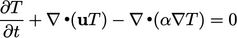(5.12)

If the Gauss-Seidel method is applied to solve the equation, a convergent solution is only guaranteed if the diagonal dominance condition of Sec. 5.3 is satisﬁed.

The contributions to the diagonal and oﬀ-diagonal coeﬃcients are summarised below for each term in the equation.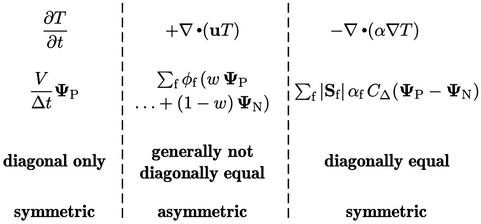The Laplacian term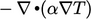(note the sign), is discretised by Eq. (3.2 ) and Eq. (3.5 ), providing a positive contribution to the diagonal coeﬃcient (relating to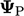) equal to the sum of the magnitude of negative oﬀ-diagonal coeﬃcients (for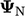).

The contribution from the advection term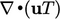, described by Eq. (3.8 ), is demonstrated above using the linear interpolation scheme of Eq. (3.4 ). The sign of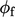depends on whether the ﬂux in incoming or outgoing from the cell. Some contributions to oﬀ-diagonal coeﬃcients are thus negative and some positive, with the diagonal contribution tending to zero as the mesh becomes more regular and ﬂow becomes more uniform.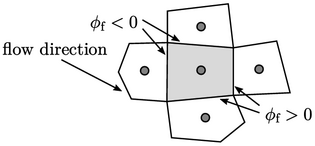Advection with linear interpolation maintains diagonal equality while its positive oﬀ-diagonal contributions oﬀset the negative contributions from the Laplacian term.

But once the advection contributions exceed the magnitude of the Laplacian contributions, diagonal equality is not achieved. This occurs when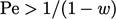(= 2 for a regular mesh), where the “numerical” Péclet number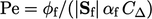is speciﬁed at cell faces from its deﬁnition in Sec. 2.21 with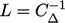.

Diagonal equality is achieved with upwind interpolation and any scheme that provides an explicit correction on upwind, e.g. linear upwind. This is conditional on mass conservation being satisﬁed, as discussed in Sec. 3.22 . If mass is not conserved, the bounded advection scheme, described in that section, is required to ensure diagonal equality.

The time derivative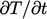increases the diagonal coeﬃcient only, so promotes diagonal dominance. Notably, the diagonal contribution of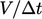from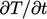becomes larger than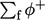from advection with upwind when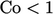.

In summary, diagonal dominance is not guaranteed due to the contribution of the advection term.

Notes on CFD: General Principles - 5.5 Diagonal dominance